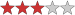## Plumb Bobs

(Eingestellt am 16. März 2021, 05:39 Uhr von Farkov)

I hope this will serve as an introduction to more difficult puzzles that have a similar theme.
I've included some hints below.

### Rules:

Normal sudoku rules apply.
All orthogonally adjacent digits are nonconsecutive.
Digits on arrows must sum to the totals in the 2-digit circles (read from top to bottom).
All orthogonally adjacent digits that sum to 5 are marked with a 'v'. Note that there are no 'v's.### Hints:

In all sudoku, as most of you probably know, all rows, columns and boxes have to sum to 45 (1+2+3+4+5+6+7+8+9). If we have an arrow with a 2-digit total occupying an entire row we know that the total must equal 45 minus the sum of the 2-digit total. So if, for example, we tried 35 as the 2-digit total the digits on the arrow would sum to 37 (45-(3+5)) which is too much, but if we tried 38 as the 2-digit total the arrow digits would only sum to 34 (45-(3+8)) which is not enough. However if we tried 36...well, I'll let you do the math :)

The nonconsecutive constraint is very handy for eliminating 2-digit totals. For example, if you know the total begins with a 2 you can automatically eliminate 21, 22, and 23 from the list of potential candidates. So if, for example, you have a 2-digit total with a 5-cell arrow on a row (ie the first 7 of the 9 cells used) and you know the total begins with a 2 you can work out the following:
21 is illegal via the nonconsecutive restraint.
22 is illegal via sudoku.
23 is illegal via the nonconsecutive restraint.
24 is viable with the 2 non-arrow cells needing to sum to 15. 6+9 is ok, but 7+8 illegal the nonconsecutive rule.
25 is viable with the 2 non-arrow cells needing to sum to 13. 4+9 is ok, but 5+8 illegal by sudoki and 6+7 by nonconsecutive.
26 is viable with the 2 non-arrow cells needing to sum to 11. 3+8 and 4+7 being ok.
27 is viable with the 2 non-arrow cells needing to sum to 9. 1+8 and 3+6 are ok.
28 is viable with the 2 non-arrow cells needing to sum to 7. 1+6 is ok.
29 seems viable, but the 2 non-arrow cells need to sum to 5. In this case 1+4 breaks the 'v' rule, and 2+3 breaks the nonconsecutive rule.

Also take note that the smallest we can make a 2-digit total on a 4-cell arrow is 15(2+3+4+6), and even that is quite problematic at times because the remaining digits are 789 which can't be placed together as they will always break the nonconsecutive rule.

Lösungscode: row 4, row 6.

Zuletzt geändert am 17. März 2021, 03:51 Uhr

Gelöst von jchan18, LeLoyJenkins, GremlinSA, piotr357, saskia-daniela, marcmees, SKORP17, kublai, MB_Cyclist, morgannamodeaura, cfop, JeremyDover, Julianl, loscpi, skywalker, bigger, nunc, ManuH, apendleton, rimodech, fuxia
Komplette Liste

### Kommentare

am 17. März 2021, 03:51 Uhr von Farkov
Fixed a silly mistake in the hints.

am 16. März 2021, 05:35 Uhr von Farkov
If you enjoy this puzzle please try my more challenging offering here: https://logic-masters.de/Raetselportal/Raetsel/zeigen.php?id=0005NA

 Schwierigkeit:Bewertung: 81 % Gelöst: 21 mal Beobachtet: 1 mal ID: 0005P9

Lösungscode:

## Anmelden